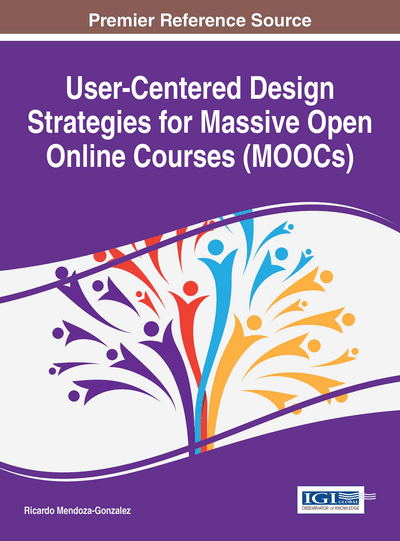Automatic Item Generation for Elementary Logic Quizzes via Markov Logic Networks

Davor Lauc (University of Zagreb, Croatia), Nina Grgić Hlača (University of Zagreb, Croatia) and Sandro Skansi (Infigo IS, Croatia)
DOI: 10.4018/978-1-4666-9743-0.ch011
Available
\$37.50
No Current Special Offers

Abstract

The aim of this chapter is to present an exam preparation system designed to generate exams for propositional logic. The main challenge was to determine a filter to single out relevant problems. An expert assessment was used to generate initial learning data for Markov Logic Network and then the result was analyzed in terms of evaluation conducted on students. The results point out that there is no significant difference (p-value of 0.2708) between problems prepared by a human examiner and problems generated.
Chapter Preview
Top

Introduction

Preparing quality exam questions and practice problems for courses at university level can be a time consuming task. This process becomes increasingly difficult if one wishes to prepare many different problems, to assure that every student has an original exam and an immense source of different practice problems.

The evolution of computational technologies has inspired many to try to resolve this problem by creating databases of practice problems (Frosini, Lazzerini, & Marcelloni, 1998). For these databases to be of practical use, they must contain a sufficient number of practice problems: although this number is not large by database standards, it is too large to be created manually. For that reason some have taken it a step further, relying on a database of templates of problems and generating a pool of original ones with the help of template variables, such as (Deane & Sheehan, 2003), (Liu, Wang, Gao, & Huang, 2005) and many others. This is not a viable path to take for generating large enough number of problems for most logical and advanced mathematical courses. Wolfram Alpha recognized the need for developing a program which could automatically generate problems in the field of mathematics and developed their recently launched Wolfram Problem Generator.

For courses in elementary physics and elementary mathematics, the main part of creating such a database is the preparation of templates, while the selection of template variables is almost trivial. On the other hand, in courses in logic and advanced mathematics, the difficult part is finding adequate values of template variables. Only small number of the possible values are valid items. In this article, a valid item is a template variable that forms a formally correct problem (e.g. in the context of propositional logic, if the exam problem is to check whether a given formula is a tautology, only well-formed formulas are considered valid items).

Moreover, only a fraction of valid items in elementary logic would be selected as interesting, hereby defined as “applicable to the real-world testing”. The most of computer generated valid items are either too trivial, in the sense that they are too easy or even when complex enough, they are too repetitive and doesn’t teach student anything of interest. Valid items that are too difficult are also not applicable to real-world testing, especially if the difficulty of problem is due to a large number of mechanical, repetitive steps required to solve the problem. For that reasons, the authors have stipulated that the desired properties of exam questions and practice problems should not only be adequate difficulty but also, somehow subjective property of “interesting question”.

The first step to preparing problems in propositional logic was to decide which formulas of propositional logic are appropriate for use in practice problems. Although it is easy to automatically generate all well-formed formulas (wffs) of propositional logic, it is not easy to automatically generate wffs of propositional logic that meet the previously stated requirements. A program that could automatically generate such propositional formulas would be a useful tool for exam authors, authors of online courses, and students, as a source of practice exercises.

This article describes an attempt to create such a program, an efficient problem generator for symbolic logic. After writing a simple program which generates well-formed formulas of propositional logic, it was obvious that only a few of the generated formulas (valid items) are suitable for use as exam questions. The next step was to generate a dataset of well-formed formulas and use it as training data to build a classifier using machine learning techniques. To enable learning from both positive and negative examples, the authors have created a dataset of formulas classified in two classes, interesting formulas (positive examples) and not interesting formulas (negative examples). Although, we could have approached this problem as a regression, marking every case with a value in the interval, the authors concluded this would make the training data more subjective.

A set of features of wffs was defined and analyzed. Using standard statistical methods, among many possible features for machine learning, the most significant features for classifying wffs as being interesting or not interesting were selected. The final classifier was developed within one of the probabilistic logic frameworks — Markov logic networks. Combining a basic program for generating wffs with the help of formal grammars and the classifier, we developed a problem generator, which was used for a few introductory logic courses. The evaluation of this model is presented in the last section.

Complete Chapter List

Search this Book:
Reset